# Civil Engineering - Soil Mechanics and Foundation Engineering

### Exercise :: Soil Mechanics and Foundation Engineering - Section 4

36.

The Terzaghi's general bearing capacity equation for a continuous footing is given by (where Nc, 2V& and Ny are bearing capacity factors.)

 A. qf = cNc + γDNq + 0.5γBNγ B. qf = cNc - γDNq + 0.5γBNγ C. qf = cNc + γDNq - 0.5γBNγ D. qf = cNc - γDNq - 0.5γBNγ

Explanation:

No answer description available for this question. Let us discuss.

37.

A clay subjected to pressure in excess to its present over-burden, is said to be

 A. pre-compressed B. pre-consolidated C. over-consolidated D. all the above.

Explanation:

No answer description available for this question. Let us discuss.

38.

The expression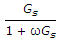ρω is used for

 A. dry density B. bulk density C. degree of saturation D. optimum water content.

Explanation:

No answer description available for this question. Let us discuss.

39.

If Cv is the coefficient of consolidation, t is the time and d is drainage path of one dimensional consolidation of soil, the time factor Tv, is given by

 A.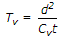B.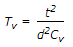C.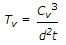D.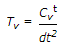Explanation:

No answer description available for this question. Let us discuss.

40.

The total active earth pressure due to dry back fill with no surcharge, acts at H/3 above the base of the wall and is directly proportional to

 A. H B.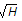C. H2 D. H3.